## 3.2.3 Perturbations to a Sample Space

As a last example of the use of perturbation ideas, consider a sample space S associated with the location (X, Y) of an object. We will assume that the object is uniformly distributed over S. Suppose that we perturb the sample space by the addition and subtraction of a term,For our development here,and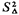must have equal area. However, the ideas can be extended to more general situations.

Now if we are interested in means and variances of some function of (X, Y), say g(X, Y), we can apply reasoning analogous to that used in the previous two sections. We assume now that the object is distributed uniformly over S'.

Exercise3.3 Defining P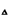appropriately, show that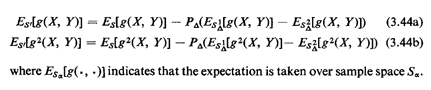Example 8: Single Facility Location

Consider the peculiarly shaped region in Figure 3.16. Assume that a facility is to be placed somewhere within the region, say at point (x0, y0). Demands for service are distributed independently and uniformly throughout the region. Then, either a server ("response unit") travels from (x0, y0) to the location of the service demand, at point (X, Y), or the person at (X, Y) travels to the facility at (x0, y0). In either case, the travel distance for a service request is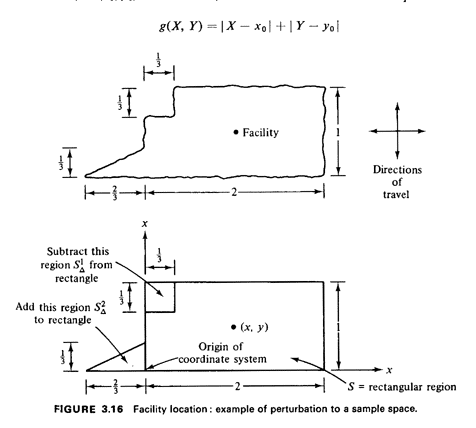We wish to locate the facility in order to minimize the expected travel distance Efg(X, Y)].

Solution The peculiar region can be thought of approximately as a rectangular region S plus the triangular regionminus the square region(see Figure 3.16). By methods discussed previously,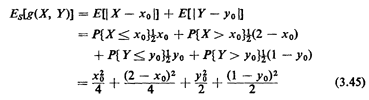By calculating areas and recognizing that the area of the rectangle is 2,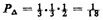To calculate the two perturbation terms, let us assume (for the moment) that x0 > 1/3 and 1/3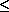y02/3; we can check our final result with these inequalities. Then, by integration or by inspection,5Taking the derivative with respect to xO and setting it equal to zero, we find that the optimal x-location is= 1, which is identical to that of the rectangle alone. Does this make intuitive sense? Taking the derivative with respect to yO and setting it equal to zero, the optimal y-location is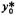= 4/9, a shift downward of about 11 percent compared to=1/2 for the rectangle. Does this make intuitive sense?

Summarizing, the location for the facility that minimizes mean travel distance to and/or from it is (= 1,=4/9) (Note that this answer satisfies our earlier inequalities since> 1/3 and 1/32/3.)

5 See See. 3.4. 1. for an "inspection method" for obtaining results such as these.